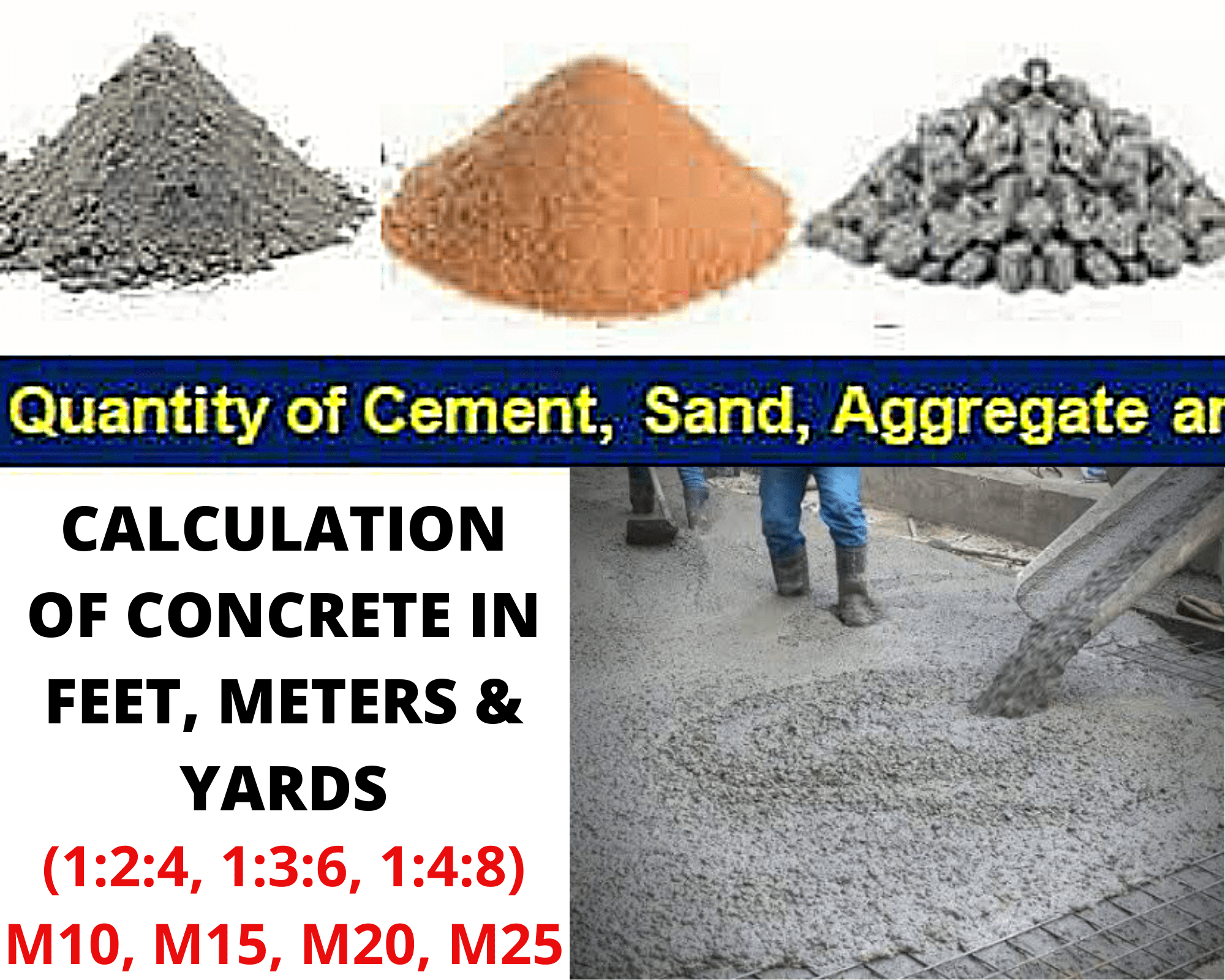Concrete calculation: How much concrete do i need?

In this article, we will discuss the concrete calculation, and you will learn how much concrete do you need and concrete yard calculation?

There’re many types of concrete and grades of concrete (e.g. Ready mix, Precast, Reinforced conc). In Calculation of concrete, first of all Multiply Length and Width & Hight to get Volume. It is same as Plaster/mortar calculation.

Concrete slab calculation:

Let suppose a slab of single story house which has 50 feet length and 25 feet width and 6″ thickness.

1. Area of slab = L×W
2. Area of slab = 50’×25′ = 1250 sft or 138.9 sqyd
3. Wet Volume of Concrete = Area × Thickness of slab
4. Wet Volume=1250×(6/12) = 625 Cft
• 6 divided by 12 to convert inch into foot

Dry Volume of concrete = 625×1.54= 962.5 Cft or 27.25 cu.m (35.31cft = 1 cu.m)

“It is compulsory to multiply with 1.54, to convert wet volume of concrete into dry volume (because addition & evaporation of water cause shrinkage in concrete mix, which ultimately decrease in volume).”

Dry volume means you need 962.5 Cft concrete for 25’x50′ slab.

Concrete yard calculation:

How to calculate yards of concrete?

There 3 most common types of concrete bags being used. E.g. 40-pound concrete bag has yield of 0.011 cubic yards, 60-pound concrete bag has yield of 0.0167 cubic yards, 80-pound concrete bag has yield of 0.0222 cubic yards.

1. Multiply dimensions in feet (L×B×H). =25×50×0.5 =625 cft
2. Multiply with 1.54 (to convert into dry volume). =962.5 cft
3. Divide by 27 to convert into Cubic Yards. =35.64 cubic yards
4. For 40-pound concrete bag, 35.64/0.011 = 3210 bags.
5. 60-pound concrete bag, 35.64/0.0167 = 2138 bags.
6. For 80-pound concrete bag, 35.64/0.0222 = 1610 bags.

Concrete bag calculation:

How many concrete bags do i need?

There are 3 types of commonly used concrete bags. Concrete mix yield increase about 0.15 about every 20 pound increment in concrete bag. E.g. 40-pound concrete bag yield is 0.30 cubic feet, 60-pound concrete bag yield is 0.45 cubic feet, 80-pound concrete bag yield is 0.60 cubic feet.

If you need 962.5 cft concrete. Simply divide total volume of concrete by the yield of concrete bag. e.g.

1. For 40-pound concrete bag, 962.5/0.30 = 3208 bags.
2. 60-pound concrete bag, 962.5/0.45 = 2138 bags.
3. For 80-pound concrete bag, 962.5/0.60 = 1604 bags.

Concrete cost calculator:

As quikrete concrete bags prices for 40-pound concrete bag is \$2.68, 60-pound concrete bag is \$3.58 and 80-pound concrete bag is \$4.48.

1. For 40-pound 3208×2.68 = \$8597.44
2. 60-pound 2138×3.58 = \$7654.04
3. For 80-pound 1604×4.48 = \$7185.92

How to calculate crushed concrete:

Now we will use dry volume of 25×50×0.5 =962.5 in crushed concrete calculation to get quantity of cement, sand & aggregate for 1:2:4 concrete mix.

Ratio of concrete mix (Grade M15) = 1:2:4

Sum of ratio = 7

Cement calculation for Concrete:

1. Quantity of cement for area 25×50×0.5 (×1.52) = (1÷7)×962.5 = 137.5 Cft
2. Cement = (1÷7)×27.25 = 3.9 Cum

Density of cement = 1440kg/m3 or 40.77kg/cft

• Multiply cft with 40.77 & cum with 1440 to get quantity of cement in KGs.

Cft>>> 137.5×40.77= 5606 Kg

Cum>>> 3.9×1440= 5606 kg

How to calculate cement bags in concrete? To find cement Bags divide by 50 (50 kg in one bag of cement).

5606/50 = 112 bags of cement

Or directly calculate cement bags from Volume:

Volume of cement bag= 0.034cum or 1.226cft

Cft>>> 137.5÷1.226 = 112 Bags

Sand calculation in Concrete:

1. Quantity of sand 25×50×0.5 (×1.52) = (2÷7)×962.5 = 275 Cft
2. Sand = (2÷7)×27.25 = 7.78 Cu.m

Density of sand is 1600kg/m3 OR 45.30 kg/cft

Crush calculation in Concrete:

1. Quantity of crush for 25×50×0.5 (×1.52) = (4÷7)×962.5 = 550Cft
2. To convert in cubic yard divide 550 by 27 = 20.3 cubic yards.
3. Convert in kgs multiply 20.3 with 764.5 = 15562 kgs.
4. To convert into tons just divide with 1000= 15.5 tons.
5. crush = (4÷7)×27.25 = 15.57 Cu.m

Calculation of Water Cement Ratio in concrete:

1. Water cement ratio means quantity of water against cement (in kgs).
2. W-C Ratio = 50% × Quantity of cement (kg)
3. Water= 5606×0.50 = 2803 liters.

50% of water quantity is commonly used in ordinary concrete mix , but it can be b/w 27% to 50% for designed mix concrete.

Concrete pier size calculator:

The simple rule for concrete pier size calculation is to add 1 inch in diameter of pier against 1 foot of span. 6 feet of span can stand on 6″ diameter of pier, 8′ can stand on 8″, 10″ can stand on 10″. If the span is more than 12 feet just add one more pier in between.

Share